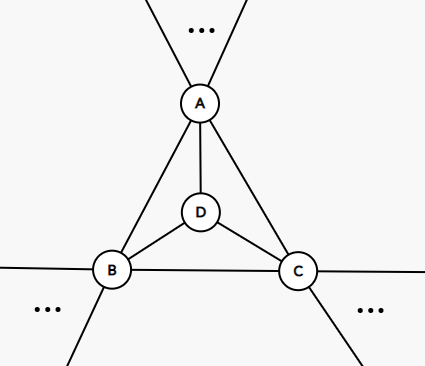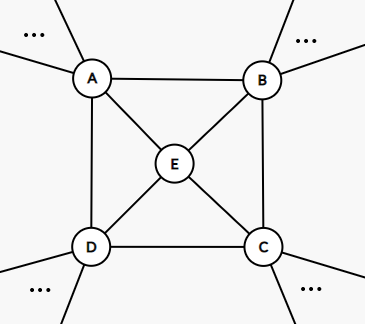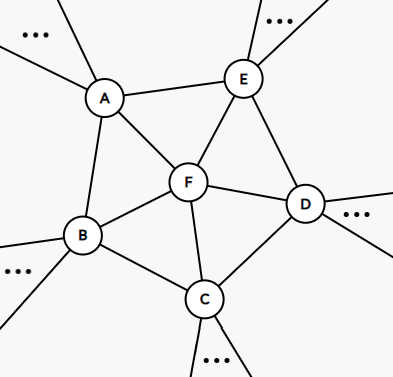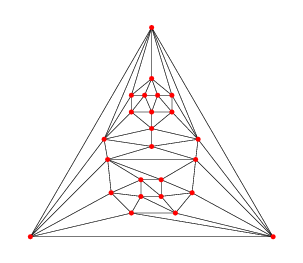## 基础知识

• 随便举个例子吧：$K_n$ 的任意 $m$ 元子集的导出子图都是 $K_m$. （当然，$m\leqslant n$ ...）

• 极大平面图又称三角剖分图，其每个面都是三角（二维单形就是三角，课上许老师没有提单形的故事，但其实单形剖分是在任何维度存在的）；
• 任何一个图都可以通过不断加边而变成一个极大平面图。

### 符号说明

• $n$：点数；
• $m$：边数；
• $\delta$：图中顶点的最小度数.

• Kempe 链：只含两种颜色的链.
• Kempe 变换：一种通过换色来减少颜色的技巧.

## Kempe 的证明思路

• 容易验证 $n\leqslant 5$ 时，所有的极大平面图都可以被 $4$-染色.
• $n\leqslant p$ 时，所有 $n$ 个顶点的极大平面图都可以被 $4$-染色，考虑 $p+1$ 的情. 由 Euler 公式可知，对于极大平面图，有 $3\leqslant \delta\leqslant 5$，故分三种情况讨论：
• $\delta=3$由于 $\delta=3$，故必定会存在一个 $3$ 度顶点，即有图中所示的结构. 记 $G'=G-D$，则 $|G'|=p$，因而可以被 $4$-染色. 而 $D$ 相邻的 $A,B,C$ 点也至多占掉三种颜色，故此时的图 $G$ 是可以被 $4$-染色的.
• $\delta=4$同上，会存在一个图示的结构，记 $G'=G-E$，则 $G'$ 有一个 $4$-染色. 若 $A,B,C,D$ 只用了至多 $3$ 种颜色，则可以完全沿用上述方法. 因此只讨论 $A,B,C,D$ 分别用了颜色 $1,2,3,4$ 的情形. 此时采用的办法被称为 Kempe 变换
考虑 $G'$ 中，颜色 $1$$3$ 的导出子图 $G'[1,3]$
• $A$$C$ 不在 $G'[1,3]$ 的同一个连通分支内，则可以将其中一者（不妨设是 $A$）所在的连通分支上的 $1$$3$ 全部交换，该种转换不会产生颜色的冲突. 转换完毕后，$A$$C$ 将变为同色，即 $A,B,C,D$ 只占三种颜色；
• $A$$C$$G'[1,3]$ 的同一连通分支内，则必定存在一条连接 $A$$C$$1-3-1-3-\cdots$ 路径（这样的路径称为 Kempe 链）. 此时考虑 $G'[2,4]$，则 $B$$D$ 一定不在 $G'[2,4]$ 的同一连通分支内（有点像 Jordan 闭曲线定理的那种感觉，$B$$D$ 一个在内一个在外），即可以对 $G'[2,4]$ 做变换，此时也可转换为 $A,B,C,D$ 只占三种颜色的情形.
• $\delta=5$同样会存在一个图示的结构，记 $G'=G-F$，则 $G'$ 存在一个 $4$-染色. 若 $A,B,C,D,E$ 只用了至多 $3$ 种颜色，则很容易给出该种情形的一个 $4$-染色. 当 $A,B,C,D,E$ 恰好用了 $4$ 种颜色时，必定有且仅有两个顶点同色，不失一般性，设 $f(A)=1,f(B)=f(E)=2,f(C)=3,f(D)=4$. 此时考虑 $G'[1,3]$$G'[1,4]$
• $A,C$$A,D$ 至少有一对在相应的导出子图上不连通，则可以直接采取换色的方式消灭掉颜色 $1$，此时在 $G$ 中给 $F$ 染颜色 $1$ 即可.
• $A,C$$A,D$ 在相应的导出子图上都连通，则在 $G'$ 中存在一条连接 $A,C$$1-3-1-3\cdots$ 链和一条连接 $A,D$$2-4-2-4\cdots$ 链，分别将 $B$$E$ 包围. 故类似 $\delta=4$ 的情况，不难发现此时不存在 $E\to C$$B\to C$ 的 Kempe 链，因而进行两次换色，即可将颜色 $2$ 消除. 进一步给 $F$ 染颜色 $4$ 即可得到 $G$$4$-染色.

$\delta=5$，且 $|f\{A,B,C,D,E\}|=4$，且 $A,C$$G'[1,3]$ 中连通，$A,D$$G'[1,4]$ 中连通时，我们需要进行两次换色：

1. $G'[2,3]$ 中，将 $E$ 所在的连通分支中的所有 $2$$3$ 对换，即可将 $E$ 换为颜色 $3$.
2. $G'[2,4]$ 中，将 $B$ 所在的连通分支中的所有 $2$$4$ 对换，即可将 $B$ 换为颜色 $4$.## 对 Kempe 工作的进一步思考

Mr. Xu 的猜想！ 对于 $\delta=5$ 的情形，对任意一个 $5$ 度顶点 $v_0$$N(v_0)=\{v_1,v_2,v_3,v_4,v_5\}$），都存在 $G'$ 的一种染色方案 $f$，s.t. 存在 $v_1\to v_3$$v_1\to v_4$ 两条 Kempe 链，且这两条链仅在 $v_1$ 相交.

• 貌似 Heawood 的图中，中心的 $5$ 度顶点就根本不行...
• 我始终觉得这个猜想只需要存在性就行了（存在一个 $5$ 度顶点...），但 Mr. Xu 课上说的的确是任意... 迷惑...